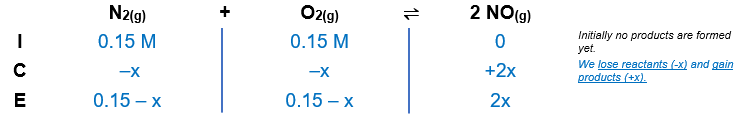# Problem: Consider the reaction:    N2(g) + O2(g) ⇌ 2 NO(g)for which Kc = 0.10 at 2,000 °C. Starting with initial concentrations of 0.15 M N2 and 0.15 M O2, determine the equilibrium concentration of NO.A. 0.020 M B. 0.13 M C. 0.0071 M D. 0.014 ME. 0.041 M

###### FREE Expert Solution

We’re being asked to calculate the equilibrium concentration of NO, given the following reaction:

N2(g) + O2(g) ⇌ 2 NO(g);  Kc = 0.10

We're given the initial concentrations of the reactants:

[N2] = 0.15 M
[O2] = 0.15 M

From this, we can construct an ICE table:The Kc expression for the reaction is:

85% (434 ratings)###### Problem Details

Consider the reaction:    N2(g) + O2(g) ⇌ 2 NO(g)
for which Kc = 0.10 at 2,000 °C. Starting with initial concentrations of 0.15 M N2 and 0.15 M O2, determine the equilibrium concentration of NO.

A. 0.020 M

B. 0.13 M

C. 0.0071 M

D. 0.014 M

E. 0.041 M